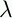September 22, 1999 Perl and the Lambda Calculus Slide #28

# Recursion

• We want

```        ADD =mn.(IF (IS_ZERO m) n (ADD (PRED m) (SUCC n)))
```
• First, a brief digression

• If f is a function, and f(x) = x, we say x is a fixed point of f

• Example: 0 is a fixed point for the `square' function

• Consider this function:

```        R =g.mn.(IF (IS_ZERO m) n (g (PRED m) (SUCC n)))
```
• R transforms some function g in a rather bizarre way

• But notice that if ADD is the addition function we desire, then

```        (R ADD) == ADD
```
• So the elusive addition function is a fixed point of R

 NextCopyright © 1999 M-J. Dominus